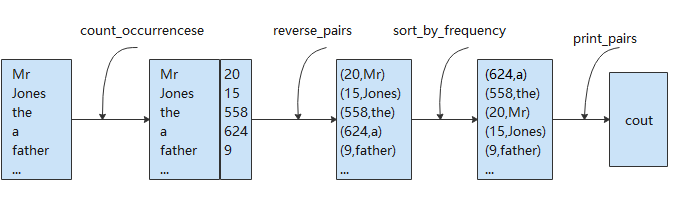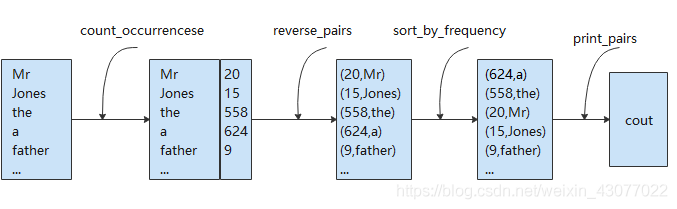10 篇文章 0 订阅

# Modern C++ 学习笔记——C++函数式编程

## 函数式编程

### 什么是函数式编程？

f(x) = 2x^2 +x+3
g(x) = 3f(x) + 5 = 6x^2 + 3x + 14
h(x) = f(x) + g(x) = 8x^2 + 4x + 17


• 无状态：函数不维护任何状态。函数式编程的核心精神是stateless
• 不可变数据输入数据不可变，动了输入数据就有危险，所以要返回新的数据集。
或许这么说有点干燥，为了更好的帮助理解，再举一个最简单的例子：
int copy_add(int x, int y)
{
return x + y;
}
{
x += y;
return x;
}


Functional programming is a style of programming that emphasizes the evaluation of expressions, rather than execution of commands. The expressions in these languages are formed by using functions to combine basic values. A functional language is a language that supports and encourages programming in a functional style.

### 函数式编程

void print_word(vector<string>& words) {
unordered_map<string, int> wordCount;
for (auto&& s : words) {
unordered_map<string, int>::iterator iter = wordCount.find(s);
if (iter == wordCount.end()) {
wordCount.insert({s, 1});
} else {
wordCount[s]++;
}
}
vector<pair<int, string>> reverseword;
for (auto it = wordCount.begin(); it != wordCount.end(); ++it) {
reverseword.emplace_back(make_pair(it->second, it->first));
}
sort(reverseword.begin(), reverseword.end(), [](const pair<int, string>& lhs, const pair<int, string>& rhs) {
return lhs.first > rhs.first;
}); // 高阶函数
for (auto& p : reverseword) {
cout << "word is " << p.second << ", count is " << p.first << endl;
}
}


• 将所有的单词插入unordered_map<string, int>中，并统计出现的次数（unordered_map要比map更快些）
vector< string> -> unordered_map<string, int>
• 将unordered_map<string, int>中的键值对转换为pair<int, string>并存入vector
unordered_map<string, int> ->vector<pair<int, string>>
• 对所有pair<int, string>排序
vector<pair<int, string>> -> vector<pair<int, string>>
• 遍历vector，输出单词和统计次数
vector<pair<int, string>> -> voidunordered_map<string, int> count_occurrencese(vector<string> items);
vector<pair<int, string>> reverse_pairs(unordered_map<string, int> pairs);
vector<pair<int, string>> sort_by_frequency(vector<pair<int, string>>);
void print_pairs(vector<pair<int, string>> pairs);


void print_words(vector<string>& words)
{
return print_pairs(
sort_by_frequency(
reverse_pairs(
count_occurrencese(words)
)
);
}


template <typename C, typename T = typename C::value_type>
std::unordered_map<T, unsigned int> count_occurrences(const C& collection)
template <typename C, typename P1, typename P2>
std::vector<std::pair<P2, P1>> reverse_pairs(const C& collection);


### 函数式编程的特点

• 会影响函数结果的只是函数的参数，没有对环境的依赖。
• 返回的结果就是函数执行的唯一结果，不产生对环境的其他影响。
• 函数的执行没有顺序上的问题
• 函数可以像普通的对象一样被传递、使用或返回。
• 代码更像是说明式而非命令式。熟悉函数式编程后，你会发现说明式代码的可读性比命令式更高，代码更短，可复用性更高。
• 无状态，没有状态就没有伤害，就像没有依赖就没有伤害一样。

## 函数式编程用到的技术

### first class function（头等函数）

class filter{ // 函数对象
public:
students()
{
names.insert("abc");
names.insert("John");
}
bool operator()(std::string name) {
return names.find(name) == names.end();
}
private:
set<std::string> names;
};
// lambda 表达式，用auto捕获匿名函数对象
auto add2 = [](int x) {	return x + 2; }


### map & reduce & filter

#### map

Map在C++中的直接映射是transform（头文件< algorithm>）。他所做的事情也是数学上的映射，把一个范围里的对象转换为相同数量的另外一些对象。假如有类person，我需要获得人到姓名的映射，即vector<person> -> vector<string>

struct person;
vector<string> GetNames(vector<person> people)
{
vector<string> names;
transform(people.begin(), people.end(), back_inserter(names), [](const person& tmp) {
return tmp.name;
});
return names;
}


std::back_inserter是定义在头文件 <iterator>中，用于构造 std::back_insert_iterator 的便利函数模板.

#### reduce

Reduce在C++中的直接映射是accumulate（头文件< numeric>）。它的功能是在指定的范围内，使用给定的出事和函数对象，从左到右对数值进行归并。看两个计算平均值的写法：

double average_score_1(const vector<int>& scores)
{
int sum = 0;
for (int socre : scores) {
sum += socre;
}
return sum / (double)scores.size();
}

double average_score_2(const vector<int>& scores)
{
return accumulate(scores.begin(), scores.end(), 0) / (double)scores.size();
}


	int product = std::accumulate(v.begin(), v.end(), 1, std::multiplies<int>());


int main()
{
std::vector<double> v(10'000'007, 0.5);
{
auto t1 = std::chrono::high_resolution_clock::now();
double result = std::accumulate(v.begin(), v.end(), 0.0);
auto t2 = std::chrono::high_resolution_clock::now();
std::chrono::duration<double, std::milli> ms = t2 - t1;
std::cout << std::fixed << "std::accumulate result " << result
<< " took " << ms.count() << " ms\n";
}
{
auto t1 = std::chrono::high_resolution_clock::now();
double result = std::reduce(std::execution::par, v.begin(), v.end());
auto t2 = std::chrono::high_resolution_clock::now();
std::chrono::duration<double, std::milli> ms = t2 - t1;
std::cout << "std::reduce result "
<< result << " took " << ms.count() << " ms\n";
}
}


std::accumulate result 5000003.50000 took 12.7365 ms
std::reduce result 5000003.50000 took 5.06423 ms


#### filter

Filter的功能是进行过滤，筛选出符合条件的成员。在C++中的映射有copy_if和partition。

auto is_female = [](const person& tmp) { return tmp.female; };
auto iter = std::partition(people.begin(), people.end(), is_female);

vector<person> females;
std::copy_if(people.cbegin(), people.cend(), std::back_inserter(females), is_female);


### pipeline（管道）

void print_words(vector<string>& words)
{
return print_pairs(
sort_by_frequency(
reverse_pairs(
count_occurrencese(words)
)
);
}


pipeline 管道借鉴于Unix Shell的管道操作——把若干个命令串起来，前面命令的输出成为后面命令的输入，如此完成一个流式计算。（注：管道绝对是一个伟大的发明，他的设哲学就是KISS – 让每个功能就做一件事，并把这件事做到极致，软件或程序的拼装会变得更为简单和直观。）

ps auwwx | awk '{print \$2}' | sort -n | xargs echo


C++20引入范围库（ranges）之后，可以使用operator |链接两个范围适配器闭包对象的结果。而在此之前我们可以尝试重载管道符，达到类似的效果。以下例子仅为了说明该项技术。

template<typename T, typename F>
auto operator | (T t, F f) -> T
{
return f(t);
}
auto f = [](const int& a) {return a + 1;};
auto g = [](const int& a) {return a * 2;};
auto h = [](const int& a) {return a - 1;};
auto y = 3 | h | g | f;


### currying (柯里化)

auto addThree = [](int x, int y, int z){
return x + y + z; };
return [=](int z) {
};
};
return [=](int y, int z) {
};
};
return [=](int y) {
};
};



addOneByOne(1)(2)(4)，而这个过程就被称作currying（柯里化）。### recursing & tail recursion optimization (递归&尾递归优化)

C++ 标准库并不保证尾递归优化能够执行，但是主流的C++编译器（如GCC\Clang\MVSC）都是支持尾递归优化的。

《Functional Programming in C++》
https://www.manning.com/books/functional-programming-in-c-plus-plus

https://zh.cppreference.com/w/cpp/algorithm/transform

https://zh.cppreference.com/w/cpp/iterator/back_inserter

https://zh.cppreference.com/w/cpp/algorithm/accumulate

https://zh.cppreference.com/w/cpp/algorithm/reduce

https://zh.cppreference.com/w/cpp/algorithm/execution_policy_tag_t

https://zh.cppreference.com/w/cpp/algorithm/partition

https://zh.cppreference.com/w/cpp/ranges

How can currying be done in C++?
https://stackoverflow.com/questions/152005/how-can-currying-be-done-in-c

https://coolshell.cn/articles/10822.html

07-022807-0114
05-22100
06-2528
05-2650
01-1183
07-219
07-2013
07-1711
07-1818
07-1212
07-1989
05-19863
01-14448
07-1510
07-1611
08-1755点击重新获取扫码支付余额充值